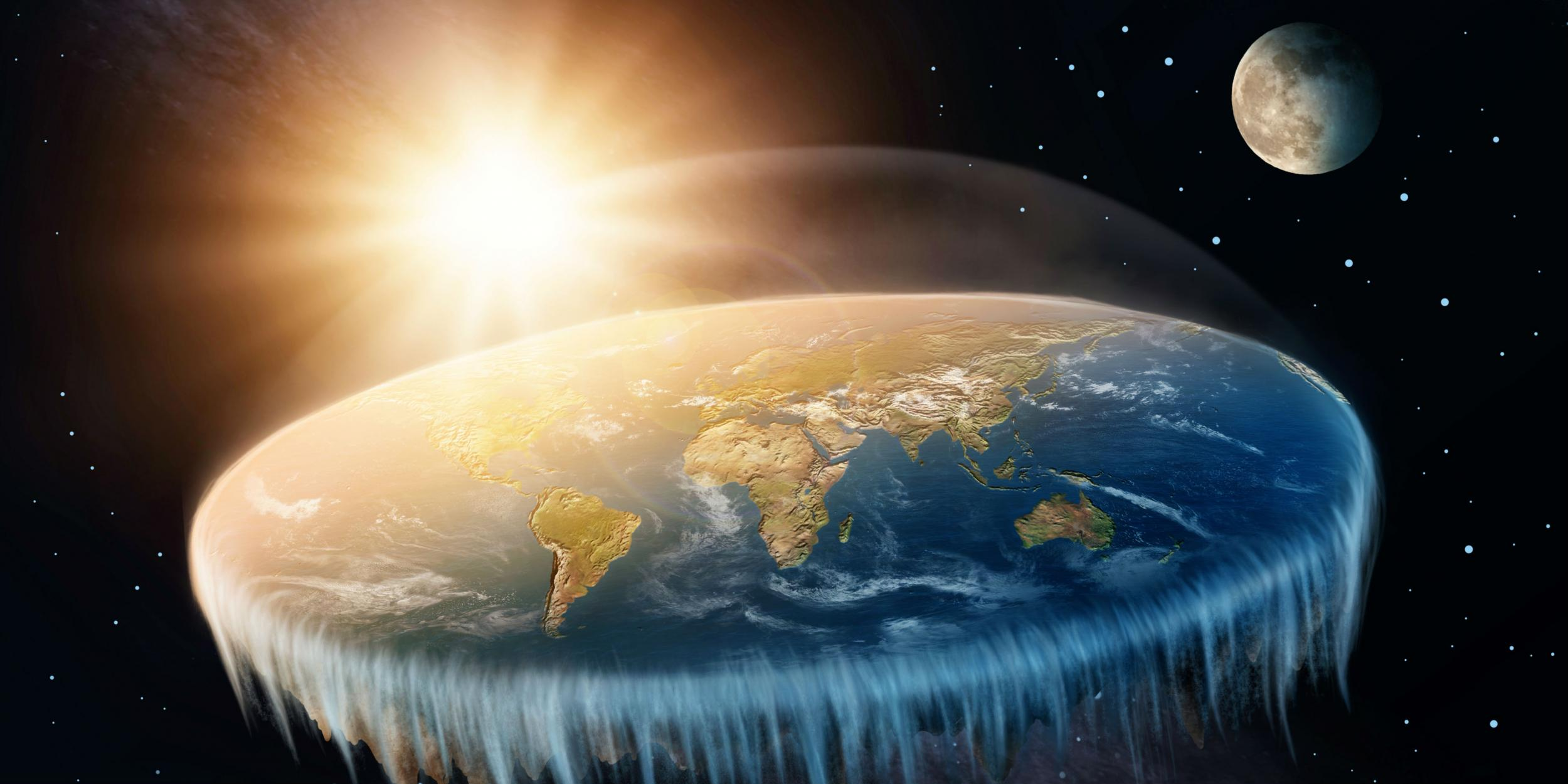# Gravity in Space

An intriguing phenomenon that took millennia for the modern human to explain is gravity. First explained by Sir Isaac M. Newton, gravity as a force as a function of mass is a difficult one for many to wrap their heads around. The reason many non-scientists struggle to understand the basics of gravity is because it only comes into play with extremely massive bodies. For example, electrostatic and magnetic forces can move even tiny atoms, but gravity does not begin to have a noticeable effect on bodies until they are massive. Furthermore, even the “weak” nuclear forces that hold atoms together are 10 to the 25th times more powerful than gravity [read more here].

How, then, does our planet orbit a sun 90 million miles away from us if it’s only bound by such an insignificant force of attraction? The answer lies in the math behind gravity. The force due to gravity between two objects can be described by a single equation: F = G*(m1m2)/r^2, where G is a constant, m1/m2 are the masses of the bodies, and r is the distance between the two bodies. Analyzing this formula reveals a very delicate balance between mass and distance. As the two objects separate, the force due to gravity becomes exponentially smaller. But as the objects’ masses increase linearly, the total force due to gravity increases exponentially. This helps to explain why our planet, though so far away from the Sun, is still tethered to the sun–because both the sun and the Earth are incomprehensibly massive.Here is a photo depicting the “flat Earth” conspiracy theory, one that is inconsistent with gravity and most other scientific findings. Independent.co.uk